# Online Verbal Reasoning Test - Verbal Reasoning Test 7Loading Test...

Instruction:

• Total number of questions : 20.
• Time alloted : 30 minutes.
• Each question carry 1 mark, no negative marks.
• DO NOT refresh the page.
• All the best :-).

1.

One morning Udai and Vishal were talking to each other face to face at a crossing. If Vishal's shadow was exactly to the left of Udai, which direction was Udai facing?

A.
 EastB.
 WestC.
 NorthD.
 SouthCorrect Answer: Option C

Explanation: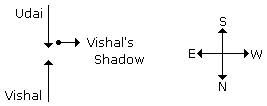Learn more problems on : Direction Sense Test

2.

Radha moves towards South-East a distance of 7 km, then she moves towards West and travels a distance of 14 km. From here she moves towards North-West a distance of 7 km and finally she moves a distance of 4 km towards east. How far is she now from the starting point?

A.
 3 kmB.
 4 kmC.
 10 kmD.
 11 kmCorrect Answer: Option C

Explanation: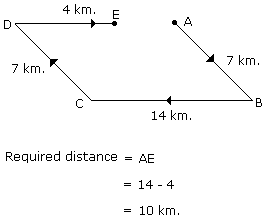Learn more problems on : Direction Sense Test

3.

From his house, Lokesh went 15 km to the North. Then he turned west and covered 10 km. Then he turned south and covered 5 km. Finally turning to the east, he covered 10 km. In which direction is he from his house?

A.
 EastB.
 WestC.
 NorthD.
 SouthCorrect Answer: Option C

Explanation: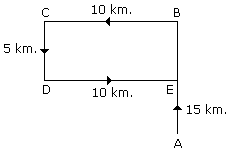Therefore, it is clear that he is in the North from his house.

Learn more problems on : Direction Sense Test

4.

Pointing to a photograph of a boy Suresh said, "He is the son of the only son of my mother." How is Suresh related to that boy?

A.
 BrotherB.
 UncleC.
 CousinD.
 FatherCorrect Answer: Option D

Explanation:

The boy in the photograph is the only son of the son of Suresh's mother i.e., the son of Suresh. Hence, Suresh is the father of boy.

Learn more problems on : Blood Relation Test

5.

Introducing a man, a woman said, "He is the only son of the mother of my mother." How is the woman related to the man?

A.
 MotherB.
 SisterC.
 NieceD.
 Maternal auntCorrect Answer: Option C

Explanation:

The man is the only son of the mother of the woman. Hence, the man is the maternal uncle of the woman. So, the woman is the niece of the man.

Learn more problems on : Blood Relation Test

6.

Pointing to a boy in the photograph Reena said, "He is the only son of the only child of my grandfather." How Reena is related to that boy?

A.
 MotherB.
 SisterC.
 AuntD.
 Cannot be determinedCorrect Answer: Option B

Explanation:

The boy in the photograph is the only son of Reena's grandfather's only son; i.e., the boy is the only son of Reena's father.

Hence, the boy is the brother of Reena or Reena is the sister of the boy.

Learn more problems on : Blood Relation Test

7.

Which one of the following is always found in 'Phrase'?

A.
 NomenclatureB.
 ManifestationC.
 Pictorial effectD.
 GlossaryCorrect Answer: Option D

Learn more problems on : Verification of Truth

Direction (for Q.No. 8):

Six dice with upper faces erased are as shows.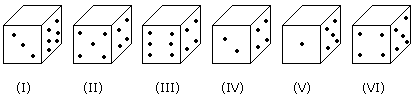The sum of the numbers of dots on the opposite face is 7.

8.

If the dice (I), (II) and (III) have even number of dots on their bottom faces, then what would be the total number of dots on their top faces?

A.
 7B.
 11C.
 12D.
 14Correct Answer: Option B

Explanation:

No. of dots on the top faces of dice (I), (II) and (III) are 5, 1 and 5 respectively.

Required total = 5 + 1 + 5 = 11

Direction (for Q.No. 9):

Below in each of the questions are given two statements I and II. These statements may be either independent causes or may be effects of independent causes or a common cause. One of these statements may be the effect of the other statements. Read both the statements and decide which of the following answer choice correctly depicts the relationship between these two statements.

• (A) If statement I is the cause and statement II is its effect.
• (B) If statement II is the cause and statement I is its effect.
• (C) If both the statements I and II are independent causes.
• (D) If both the statements I and II are effects of independent causes.
• (E) If both the statements I and II are effects of some common cause.
9.

Statements:

1. Police had resorted to lathi-charge to disperse the unruly mob from the civic headquarters.
2. The civic administration has recently hiked the property tax of the residential buildings by about 30 percent.

A.
 Statement I is the cause and statement II is its effect.B.
 Statement II is the cause and statement I is its effect.C.
 Both the statements I and II are independent causes.D.
 Both the statements I and II are effects of independent causes.E.
 Both the statements I and II are effects of some common cause.Correct Answer: Option D

Explanation:

Both the statements I and II are the effects of independent causes.

Learn more problems on : Cause and Effect

10.

In the figure given below, square represents doctors, triangle represents ladies and circle represents surgeon. By which letter the ladies who doctor and surgeon both are represented ?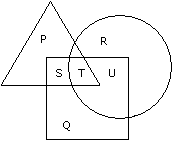A.
 UB.
 TC.
 SD.
 PCorrect Answer: Option B

Learn more problems on : Venn Diagrams

11.

Study the diagram and identify the people who can speak only one language.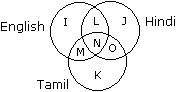A.
 L + M + OB.
 K + J + IC.
 KD.
 ICorrect Answer: Option B

Explanation:

The regions represented by the letters K, J and I denote such people who can speak only one language.

Learn more problems on : Venn Diagrams

Direction (for Q.No. 12):

Study the following figure and answer the questions given below.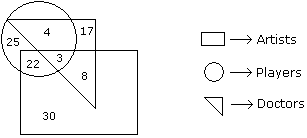12.

How many artists are players ?

A.
 5B.
 8C.
 25D.
 16Correct Answer: Option C

Explanation:

The number of artists who are players is 22 + 3 = 25.

Learn more problems on : Venn Diagrams

Direction (for Q.No. 13):
In each of the following questions find out the alternative which will replace the question mark.
13.

Tiger : Forest :: Otter : ?

A.
 CageB.
 SkyC.
 NestD.
 WaterCorrect Answer: Option D

Explanation:

As Tiger is found in Forest similarly Otter is found in the water.

14.

'Nun' is related to 'Convent' in the same way as 'Hen' is related to:

A.
 NestB.
 ShedC.
 CellD.
 CoteCorrect Answer: Option D

Explanation:

As dwelling place of 'Nun' is 'Convent' similarly the dwelling place of 'Hen' is 'Cote'.

Direction (for Q.No. 15):
In an Exhibition seven cars of different companies - Cadillac, Ambassador, Fiat, Maruti, Mercedes, Bedford and Fargo are standing facing to east in the following order :
1. Cadillac is next to right of Fargo.
2. Fargo is fourth to the right of Fiat.
3. Maruti car is between Ambassador and Bedford.
4. Fiat which is third to the left of Ambassador, is at one end.
15.

Which of the cars are on both the sides of cadillac car ?

A.
 Ambassador and MarutiB.
 Maruti and FiatC.
 Fargo and MercedesD.
 Ambassador and FargoCorrect Answer: Option C

Explanation: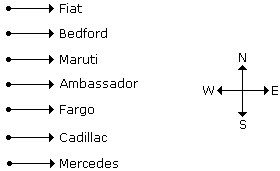Fargo and Mercedes are on both the sides of cadillac car.

Learn more problems on : Seating Arrangement

Direction (for Q.No. 16):
Six friends P, Q, R, S, T and U are sitting around the hexagonal table each at one corner and are facing the centre of the hexagonal. P is second to the left of U. Q is neighbour of R and S. T is second to the left of S.
16.

Which one is sitting opposite to P ?

A.
 RB.
 QC.
 TD.
 SCorrect Answer: Option D

Explanation: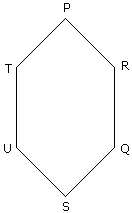S is sitting opposite to P.

Learn more problems on : Seating Arrangement

17.

Which one will replace the question mark ?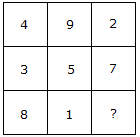A.
 9B.
 6C.
 15D.
 14Correct Answer: Option B

Explanation:

(4 + 9 + 2) = (3 + 5 + 7) = (8 + 1 + 6)

Total in each case = 15.

Learn more problems on : Character Puzzles

18.

Which one will replace the question mark ?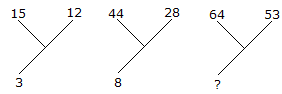A.
 30B.
 13C.
 70D.
 118Correct Answer: Option B

Explanation:

(15 + 12)/9 = 3

and (44 + 28)/9 = 8

Therefore, (64 + 53)/9 = 13.

Learn more problems on : Character Puzzles

Direction (for Q.Nos. 19 - 20):
In each of the following questions, arrange the given words in a meaningful sequence and thus find the correct answer from alternatives.
19.

Arrange the words given below in a meaningful sequence.

 1. Police 2. Punishment 3. Crime 4. Judge 5. Judgement

A.
 3, 1, 2, 4, 5B.
 1, 2, 4, 3, 5C.
 5, 4, 3, 2, 1D.
 3, 1, 4, 5, 2Correct Answer: Option D

Explanation:

The correct order is :

 Crime Police Judge Judgement Punishment 3 1 4 5 2

Learn more problems on : Logical Sequence of Words

20.

Arrange the words given below in a meaningful sequence.

 1. Rainbow 2. Rain 3. Sun 4. Happy 5. Child

A.
 4, 2, 3, 5, 1B.
 2, 3, 1, 5, 4C.
 4, 5, 1, 2, 3D.
 2, 1, 4, 5, 3Correct Answer: Option B

Explanation:

The correct order is :

 Rain Sun Rainbow Child Happy 2 3 1 5 4

Learn more problems on : Logical Sequence of Words